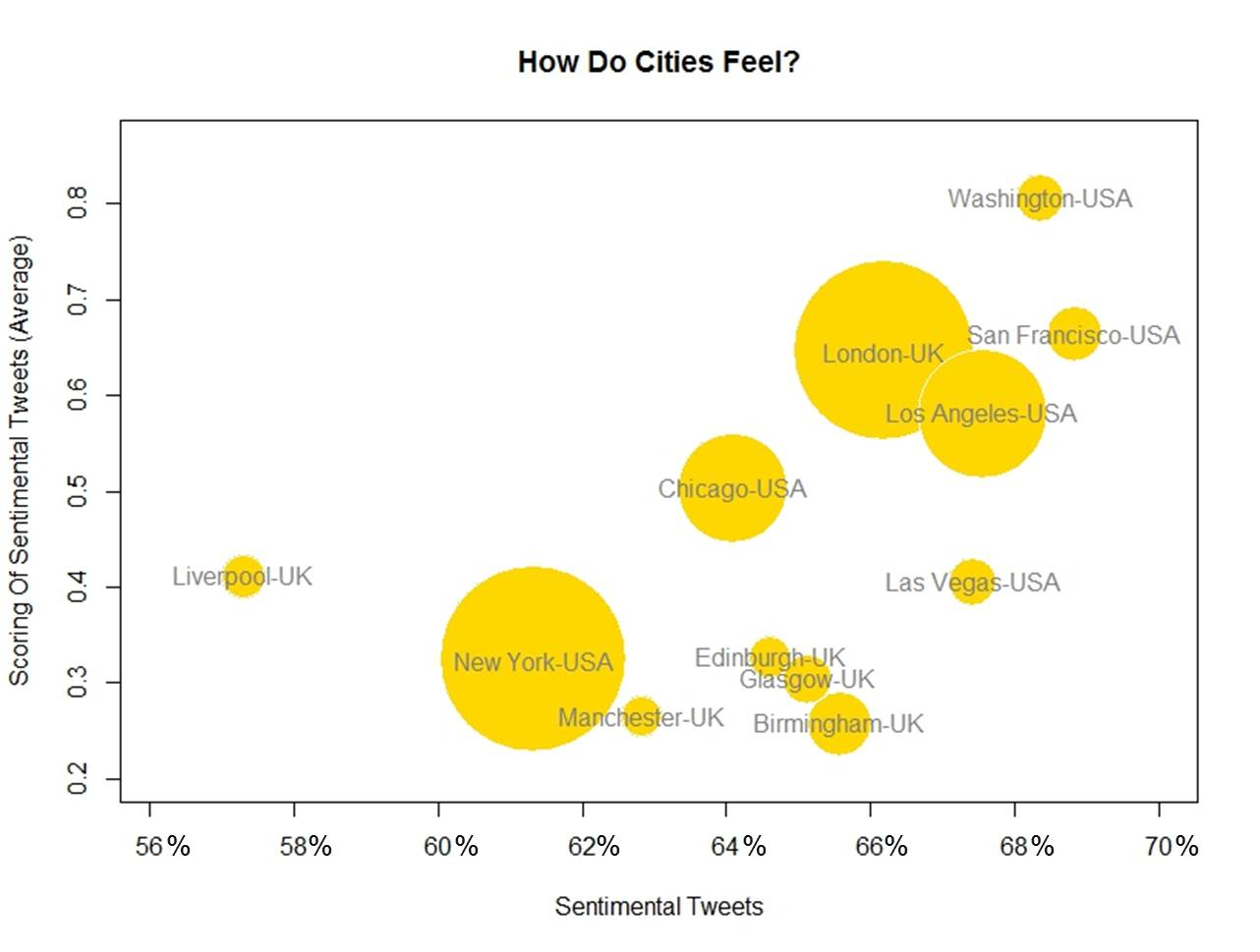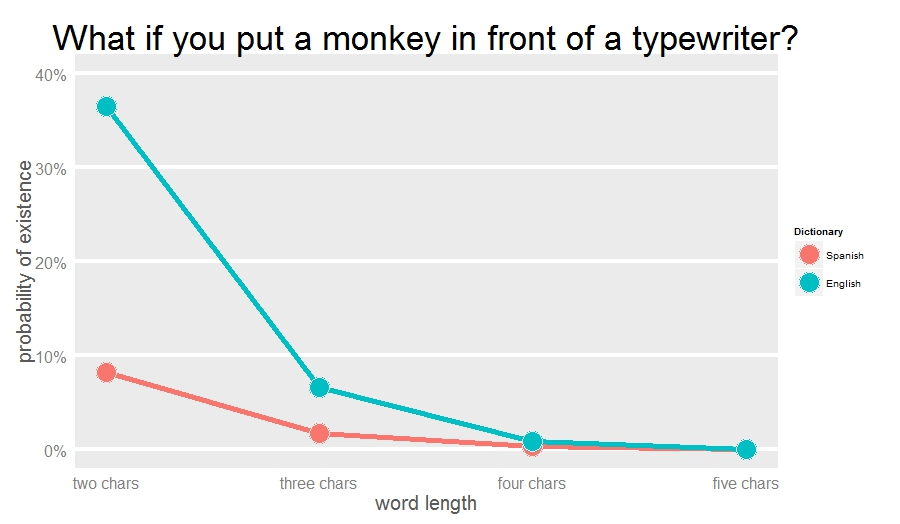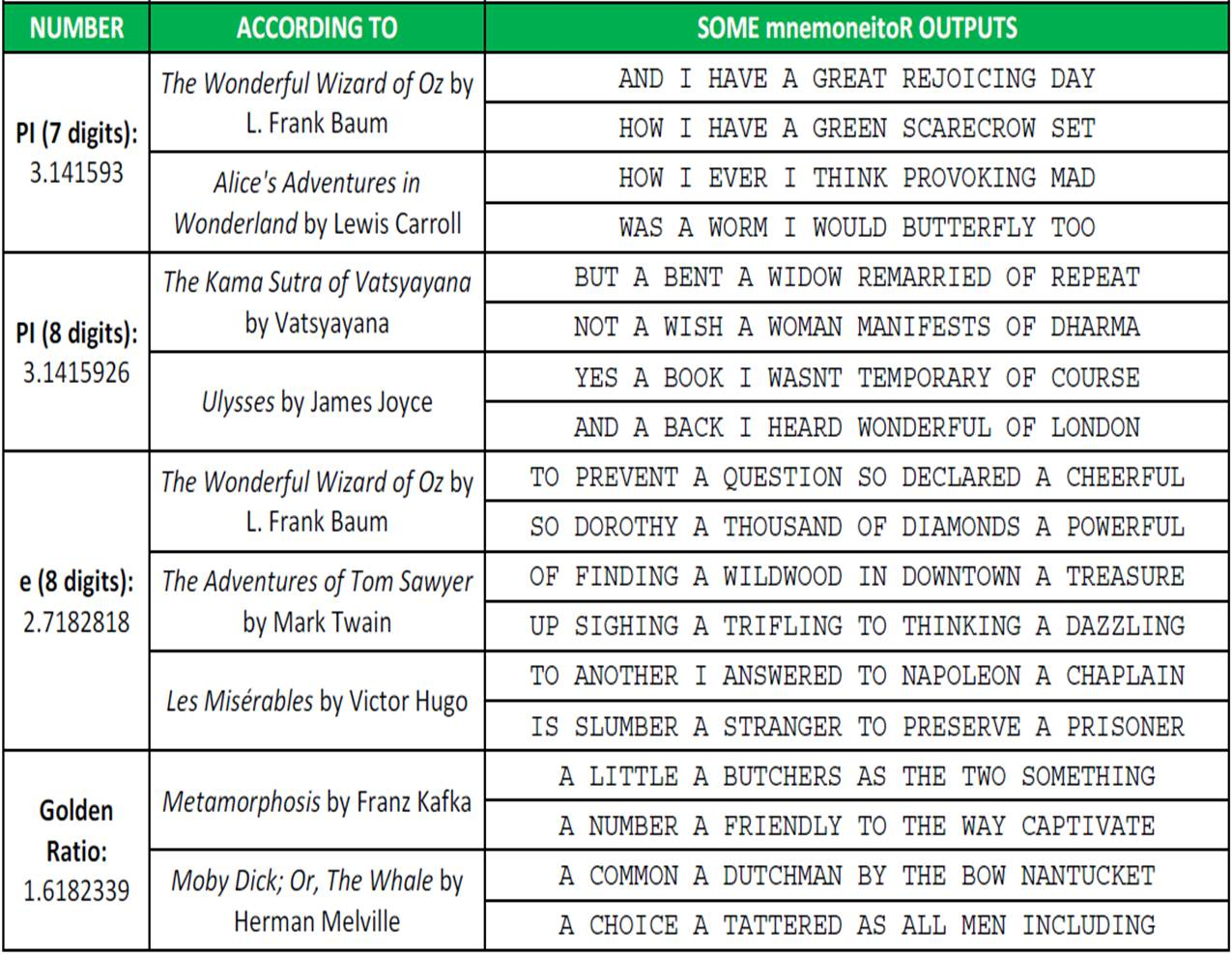# How Do Cities Feel?

If you are lost and feel alone, circumnavigate the globe (For You, Coldplay)

You can not consider yourself a R-blogger until you do an analysis of Twitter using `twitteR `package. Everybody knows it. So here I go.

Inspired by the fabulous work of Jonathan Harris I decided to compare human emotions of people living (or twittering in this case) in different cities. My plan was analysing tweets generated in different locations of USA and UK with one thing in common: all of them must contain the string “I FEEL”. These are the main steps I followed:

• Locate cities I want to analyze using world cities database of `maps` package
• Download tweets around these locations using `searchTwitter` function of `twitteR` package.
• Cross tweets with positive and negative lists of words and calculate a simple scoring for each tweet as number of positive words – number of negative words
• Calculate how many tweets have non-zero scoring; since these tweets put into words some emotion I call them sentimental tweets
• Represent cities in a bubble chart where x-axis is percentage of sentimental tweets, y-axis is average scoring and size of bubble is population

This is the result of my experiment:These are my conclusions (please, do not take it seriously):

• USA cities seem to have better vibrations and are more sentimental than UK ones
• Capital city is the happiest one for both countries
• San Francisco (USA) is the most sentimental city of the analysis; on the other hand, Liverpool (UK) is the coldest one
• The more sentimental, the better vibrations

From my point of view, this analysis has some important limitations:

• It strongly depends on particular events (i.e. local football team wins the championship)
• I have no idea of what kind of people is behind tweets
• According to my experience, `searchTwitter `only works well for a small number of searches (no more than 300); for larger number of tweets to return, it use to give malformed JSON response error from server

Anyway, I hope it will serve as starting point of some other analysis in the future. At least, I learned interesting things about R doing it.

Here you have the code:

```library(twitteR)
library(RCurl)
library(maps)
library(plyr)
library(stringr)
library(bitops)
library(scales)
#Register
if (!file.exists('cacert.perm'))
{
}
consumerKey = "YOUR CONSUMER KEY HERE"
consumerSecret = "YOUR CONSUMER SECRET HERE"
Cred <- OAuthFactory\$new(consumerKey=consumerKey,
consumerSecret=consumerSecret,
requestURL=requestURL,
accessURL=accessURL,
authURL=authURL)
Cred\$handshake(cainfo=system.file("CurlSSL", "cacert.pem", package="RCurl"))
#Save credentials
options(RCurlOptions = list(cainfo = system.file("CurlSSL", "cacert.pem", package = "RCurl")))
#Cities to analyze
cities=data.frame(
CITY=c('Edinburgh', 'London', 'Glasgow', 'Birmingham', 'Liverpool', 'Manchester',
'New York', 'Washington', 'Las Vegas', 'San Francisco', 'Chicago','Los Angeles'),
COUNTRY=c("UK", "UK", "UK", "UK", "UK", "UK", "USA", "USA", "USA", "USA", "USA", "USA"))
data(world.cities)
cities2=world.cities[which(!is.na(match(
str_trim(paste(world.cities\$name, world.cities\$country.etc, sep=",")),
str_trim(paste(cities\$CITY, cities\$COUNTRY, sep=","))
))),]
cities2\$SEARCH=paste(cities2\$lat, cities2\$long, "10mi", sep = ",")
cities2\$CITY=cities2\$name
tweets=data.frame()
for (i in 1:nrow(cities2))
{
tw=searchTwitter("I FEEL", n=400, geocode=cities2[i,]\$SEARCH)
tweets=rbind(merge(cities[i,], twListToDF(tw),all=TRUE), tweets)
}
#Save tweets
write.csv(tweets, file="tweets.csv", row.names=FALSE)
#Import csv file
hu.liu.pos = scan('lexicon/positive-words.txt',  what='character', comment.char=';')
hu.liu.neg = scan('lexicon/negative-words.txt',  what='character', comment.char=';')
#Function to clean and score tweets
score.sentiment=function(sentences, pos.words, neg.words, .progress='none')
{
require(plyr)
require(stringr)
scores=laply(sentences, function(sentence, pos.word, neg.words) {
sentence=gsub('[[:punct:]]','',sentence)
sentence=gsub('[[:cntrl:]]','',sentence)
sentence=gsub('\\d+','',sentence)
sentence=tolower(sentence)
word.list=str_split(sentence, '\\s+')
words=unlist(word.list)
pos.matches=match(words, pos.words)
neg.matches=match(words, neg.words)
pos.matches=!is.na(pos.matches)
neg.matches=!is.na(neg.matches)
score=sum(pos.matches) - sum(neg.matches)
return(score)
}, pos.words, neg.words, .progress=.progress)
scores.df=data.frame(score=scores, text=sentences)
return(scores.df)
}
cities.scores=score.sentiment(city.tweets[1:nrow(city.tweets),], hu.liu.pos, hu.liu.neg, .progress='text')
cities.scores\$pos2=apply(cities.scores, 1, function(x) regexpr(",",x)-1)
cities.scores\$CITY=apply(cities.scores, 1, function(x) substr(x, 1, x))
cities.scores=merge(x=cities.scores, y=cities, by='CITY')
df1=aggregate(cities.scores["score"], by=cities.scores[c("CITY")], FUN=length)
names(df1)=c("CITY", "TWEETS")
cities.scores2=cities.scores[abs(cities.scores\$score)>0,]
df2=aggregate(cities.scores2["score"], by=cities.scores2[c("CITY")], FUN=length)
names(df2)=c("CITY", "TWEETS.SENT")
df3=aggregate(cities.scores2["score"], by=cities.scores2[c("CITY")], FUN=mean)
names(df3)=c("CITY", "TWEETS.SENT.SCORING")
#Data frame with results
df.result=join_all(list(df1,df2,df3,cities2), by = 'CITY', type='full')
#Plot results
inches=0.85, fg="white", bg="gold", xlab="Sentimental Tweets", ylab="Scoring Of Sentimental Tweets (Average)",
main="How Do Cities Feel?")
text(100*df.result\$TWEETS.SENT/df.result\$TWEETS, df.result\$TWEETS.SENT.SCORING, paste(df.result\$CITY, df.result\$country.etc, sep="-"), cex=1, col="gray50")
```

# Shakespeare Is More Monkey-Friendly Than Cervantes

Ford, there is an infinite number of monkeys outside who want to talk to us about this script for Hamlet they have worked out (from Episode 2 of The Hitchhiker’s Guide to the Galaxy by Douglas Adams)

Some days ago I was talking with a friend about the infinite monkey theorem which is a funny interpretation of what thinking-in-infinite can produce. The same day, in my weekly English class, my teacher said that Anglo-saxon words do tend to be short, very often monosyllabic such as function words such as to, of, from etc and everyday words such as go, see run, eat, etc.

Both things made me think that a monkey could have easier to type a Shakespeare text rather than a Cervantes one. I cannot imagine a definitive way to demonstrate this but this experiment support my hypothesis. After simulating random words of 2, 3, 4 and 5 characters I look for them in English(1) and Spanish(2) dictionaries, which I previously downloaded from here. Result: I find more random words in the English one. These are the results of my experiment:For example, around 38% of two-chars words match with English dictionary and only 9% with Spanish one. This is why I think that, in the infinite, I would be easier for a monkey to replicate a Shakespeare text than a Cervantes one.

Here you have the code:

```library(ggplot2)
library(scales)
df.lang=do.call("rbind", list(esp.dic, eng.dic))
df.lang\$WORD=tolower(iconv(df.lang\$WORD, to="ASCII//TRANSLIT"))
df.lang=unique(df.lang)
results=data.frame(LANG=character(0), OCCURRENCES=numeric(0), SIZE=numeric(0), LENGTH=numeric(0))
for (i in 2:5)
{
df.monkey=data.frame(WORD=replicate(20000, paste(sample(c(letters), i, replace = TRUE), collapse='')))
results=rbind(results, data.frame(setNames(aggregate(WORD ~ ., data = merge(df.lang, df.monkey, by="WORD"), FUN=length), c("LANG","OCCURRENCES")), SIZE=20000, LENGTH=i))
}
opt=theme(panel.background = element_rect(fill="gray92"),
panel.grid.minor = element_blank(),
panel.grid.major.x = element_blank(),
panel.grid.major.y = element_line(color="white", size=1.5),
plot.title = element_text(size = 35),
axis.title = element_text(size = 20, color="gray35"),
axis.text = element_text(size=16),
axis.ticks = element_blank(),
axis.line = element_line(colour = "white"))
ggplot(data=results, aes(x=LENGTH, y=OCCURRENCES/SIZE, colour=LANG))+
geom_line(size = 2)+
scale_colour_discrete(guide = FALSE) +
geom_point(aes(fill=LANG),size=10, colour="gray92",pch=21)+
scale_x_continuous("word length", labels=c("two chars", "three chars", "four chars", "five chars"))+
scale_y_continuous("probability of existence", limits=c(0, 0.4), labels = percent)+
labs(title = "What if you put a monkey in front of a typewriter?")+
opt + scale_fill_discrete(name="Dictionary", breaks=c("ESP", "ENG"), labels=c("Spanish", "English"))
```

(1) The English dictionary was originally compiled from public domain sources
for the amSpell spell-checker by Erik Frambach e-mail: e.h.m.frambach@eco.rug.nl
(2) The Spanish dictionary has been elaborated by Juan L. Varona, Dpto. de Matematicas y Computacion, Universidad de La Rioja, Calle Luis de Ulloa s/n, 26004 SPAIN e-mail: jvarona@siur.unirioja.es

# The mnemoneitoR

AND I HAVE A GREAT REJOICING DAY (mnemonic rule generated by mnemoneitoR for first 7 digits of Pi according to The Wonderful Wizard Of Oz)

Is there some number impossible to memorize? Do not worry, here comes mnemoneitoR: the tool that you was always looking for! With mnemoneitoR you can translate any number into an easy-to-remember phrase inspired by your favorite book. It is very easy: choose a book, enter the number and mnemoneitoR will show you as many possibilities as you want. Just choose the one you like most!

There are many webs about mnemonics in the Internet, like this one. One of my favourite menmonic devices for Pi is:

HOW I WANT A DRINK, ALCOHOLIC OF COURSE, AFTER THE HEAVY LECTURES INVOLVING QUANTUM MECHANICS

The number of letters in each word gives the respective number in the sequence (i.e., 3.14159265358979).

For professional purposes, I am learning how to manage texts in R and I discovered a very useful package called `stringr`. This is the only one I need for this experiment. The process is simple: I download a book from Project Gutenberg site, clean and split the text and do simulations on the fly of a Markov Chain generated from the words of the book. Step by step:

• Downloading the book is quite simple. You search the one you want, copy the url in the code (after line “CHOOSE YOUR FAVORITE BOOK HERE”) and no more.
• After loading the text, some easy tasks are needed: remove header and footer lines, split text into words, turn them into uppercase, remove non-text characters … typical things working with texts.
• After reading the number you want to translate, I choose a word sampling along all words with the same number of letters as the first digit with probability equal to the number of appearances. This is how I initialize the phrase. Next word are chose among the set of words which are preceded by the first one and have the same number of letters as the second digit with probability equal to number of appearances, and so on. This is a simulation on the fly of Markov Chain because I do not have to calculate the chain explicitly.
• I always translate Zero with the same word you choose. I like using “OZ” instead Zero.

Most of the phrases do not have any sense but are quite funny. Few of them have some sense and maybe with a small tweak, can change into full of meaning sentences. Here you have some samples of the output of mnemoneitoR:I like how the phrases smell like the original book. I will try to improve mnemoneitoR in the future but I can imagine some uses of this current version: message generator for fortune cookies,  a cool way to translate your telephone number into a sentence …

Here you have the code. If you discover nice outputs in your experiments, please let me know:

```library(stringr)
# CHOOSE YOUR FAVORITE BOOK HERE (Currently "The Wonderful Wizard of Oz")
TEXTFILE = "data/pg55.txt"
# Remove header and footer, concatenate all of the lines, remove non-text and double spaces chars and to upper
textfile = textfile[(grep('START OF THIS PROJECT', textfile, value=FALSE)+1:grep('END OF THIS PROJECT', textfile, value=FALSE)-1)]
textfile <- paste(textfile, collapse = " ")
textfile <- gsub("[^a-zA-Z ]","", textfile)
textfile <- toupper(textfile)
textfile <- gsub("^ *|(?<= ) | *\$", "", textfile, perl=T)
# Split file into words
textfile.words <- strsplit(textfile," ")
textfile.words.freq <- as.data.frame(table(textfile.words));
names(textfile.words.freq) <- c("word", "freq")
textfile.words.freq\$length <- apply(data.frame(textfile.words.freq[,c("word")]), 1, function(x) nchar(x))
# ENTER YOUR NUMBER HERE!!!!!!
number <- 3.1415926
number <- gsub("[^0-9]","", as.character(number))
# Define the word representing Zero
zero.word = "OZ"
fg <- as.integer(substr(number, 1, 1))
df <- textfile.words.freq[textfile.words.freq\$length==fg,]
wd <- sample(df\$word, size=1, prob=df\$freq)
phrase <- c(as.character(wd))
for (j in 2:nchar(number))
{
fg <- as.integer(substr(number, j, j)) if (fg>0)
{
lc <- as.data.frame(str_locate_all(textfile, as.vector(paste(wd, " ", sep = ""))))
lc\$char <- apply(lc, 1, function(x) substr(textfile, as.integer(x)+1+fg, as.integer(x)+1+fg))
fq <- as.data.frame(table(apply(lc[lc\$char==" ",], 1, function(x) substr(textfile, as.integer(x)+1, as.integer(x)+fg))))
if (nrow(fq)==0) fq <- data.frame(word= character(0), freq= integer(0))
names(fq) <- c("word", "freq")
fq\$length <- apply(fq, 1, function(x) nchar(gsub(" ","", x)))
fq <- fq[fq\$length==fg,]
wd <- if(nrow(fq)>0) sample(fq\$word, size=1, prob=fq\$freq)
else
{
df <- textfile.words.freq[textfile.words.freq\$length==fg,]
wd <- sample(df\$word, size=1, prob=df\$freq)
}
}
else wd <- zero.word
phrase <- c(phrase, as.character(wd))
}
print(paste(phrase, collapse = " "))
```